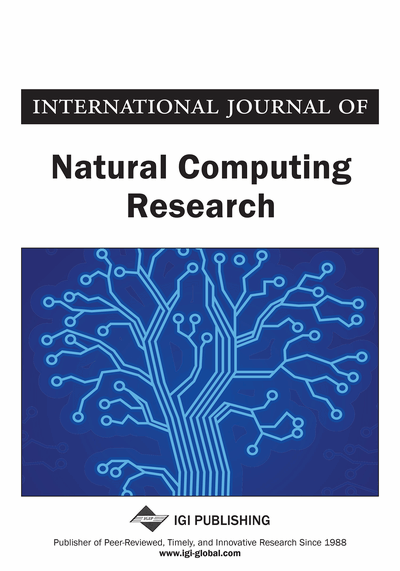# Quantum Automata with Open Time Evolution

Mika Hirvensalo
DOI: 10.4018/jncr.2010010104
OnDemand:
(Individual Articles)
Available
\$37.50
No Current Special Offers

## Abstract

In this paper, a model for finite automaton with an open quantum evolution is introduced, and its basic properties are studied. It is shown that the (fuzzy) languages accepted by open evolution quantum automata obey various closure properties. More importantly, it is shown that major other models of finite automata, including probabilistic, measure once quantum, measure many quantum, and Latvian quantum automata can be simulated by the open quantum evolution automata without increasing the number of the states.
Article Preview
Top

## 1. Introduction

### 1.1. Finite Automata

Finite automata are models of computation with a finite memory. For an exposition on finite automata, see (Eilenberg, 1974) or (Yu, 1997), for instance, but a brief description of finite automata consists of an input alphabet, the transition function, and a finite set of states, some of which are specified initial, some final. A finite automaton is deterministic, if there is only one initial state, and the transition function specifies always a single state, not a set of states. There are many variants of nondeterministic finite automata, some of which we will later treat in more detail.

The computation of a finite automaton is real time computation, meaning that the input, which is a string (called input word) over the input alphabet, is given to the automaton one letter at time. Before the computation begins, the automaton is set in an initial state, and once a letter is received, the transition function specifies how the state changes. After reading the whole input word, the state of the automaton specifies the outcome of the computation. If the input word leaves the automaton in a final state (can be also called an accepting state), then we regard the input word as accepted, otherwise rejected. The set of accepted strings is called the language accepted by the finite automaton. In general, any set of words is called a language, but languages which are accepted by deterministic finite automata, are called regular.

Hence finite automata are machines classifying words as accepted or rejected, or equivalently, machines answering to computational yes/no -questions. Restricting the computation to yes/no -problems is not a major restriction itself, as many computational problems demanding a larger output set can be relatively easily split into a sequence of yes/no -problems. On the other hand, further study (see e.g., (Eilenberg, 1974) or (Yu, 1997) shows that the deterministic finite automata are far too simple to solve any complex problems.

Nevertheless, researchers have shown interest on finite automata for many decades, despite of their weak computational power. Indeed, finite automata are not interesting because they would resolve difficult computational problems (which they do not), but because the model is very elegant and simple, bearing notable closure properties (union, intersection, complement, Kleene star) and a variety of characterizations ranging over logic and algebra (Eilenberg, 1974). Furthermore, the theory of finite automata has numerous applications in numerous fields including patter recognition, image compression, and DNA sequencing, for instance (see references in (Yu, 1997)).

The usual resource counted in finite automata computation is the number of automata states: the less, the better. In this article, we will show that the introduced variant can simulate many other models without increasing the number of states.

## Complete Article List

Search this Journal:
Reset
Volume 12: 1 Issue (2024): Forthcoming, Available for Pre-Order
Volume 11: 4 Issues (2022): 1 Released, 3 Forthcoming
Volume 10: 4 Issues (2021)
Volume 9: 4 Issues (2020)
Volume 8: 4 Issues (2019)
Volume 7: 4 Issues (2018)
Volume 6: 2 Issues (2017)
Volume 5: 4 Issues (2015)
Volume 4: 4 Issues (2014)
Volume 3: 4 Issues (2012)
Volume 2: 4 Issues (2011)
Volume 1: 4 Issues (2010)
View Complete Journal Contents Listing